#### লেখক:

Md. Manzur Rahman Ruddro

Student School of Savar

Class 10

ABSTRUCT:

The force of gravitational attraction is directly dependent upon the masses of both objects and there specific gravity and inversely proportional to the square of the distance that separates their centers.

The law is summarized symbolically as,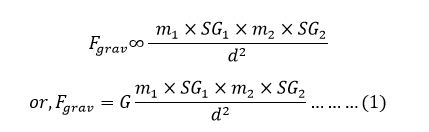Here,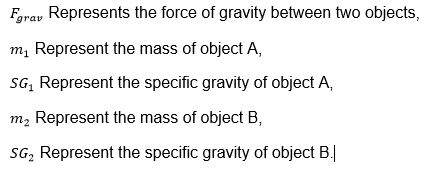And G represent Gravitational Force Constant with a value 6.673*10-11 N/m2  which is accurately determined from the results of the Cavendish experiment conducted by the British scientist Henry Cavendish in 1798 and proved by Albert Einstein.

It should be mention that the unit of force is remain Newton (N), because specific gravity have no unit,

EXPLANTION:

Let us theoretically consider two stars A and B having same mass “M”. Star A is twice denser than star B. Planet C having mass “m”, situated at the same distance “d” from two stars linearly. That means the distance between A to C is “d” as well as the distance between B to C is “d” and A, B, C are in straight line. Now here is a question. Is the gravitational force between A and C equal to the gravitational force between B and C? According to Newton's law of universal gravitation law (Newton's law of universal gravitation states that any two bodies in the universe attract each other with a force that is directly proportional to the product of their masses and inversely proportional to the square of the distance between them. It should be mention that This Gravitational Equation is valid only our Resident Universe), two forces will be same because Newton’s Gravitational force is depend upon there mass and distance between them. In these two cases, masses and distance all are same. To find the actual answer we have to understand the “Life Cycle of Star” –

LIFE CYCLE OF STAR:

A star is formed when a large amount of gas (mostly hydrogen) starts to collapse in on itself due to its gravitational attraction. As it contracts, the atoms of the gas collide with each other more and more frequently and at greater and greater speeds – the gas heats up. Eventually, the gas will be so hot that when the hydrogen atoms collide they no longer bounce off each other, but instead coalesce to form helium. The heat released in this reaction, which is like a controlled hydrogen bomb explosion, is what makes the star shine. This additional heat also increases the pressure of the gas until it is sufficient to balance the gravitational attraction, and the gas stops contracting. It is a bit like a balloon – there is a balance between the pressure of the air inside, which is trying to make the balloon expand, and the tension in the rubber, which is trying to make the balloon smaller. Stars will remain stable like this for a long time, with heat from the nuclear reactions balancing the gravitational attraction. Eventually, however, the star will run out of its hydrogen and other nuclear fuels. Paradoxically, the more fuel a star starts off with, the sooner it runs out. This is because the more massive the star is, the hotter it needs to be to balance its gravitational attraction. And the hotter it is, the faster it will use up its fuel. Our sun has probably got enough fuel for another five thousand million years or so, but more massive stars can use up their fuel in as little as one hundred million years, much less than the age of the universe. When a star runs out of fuel, it starts to cool off and so to contract. What might happen to it then was first understood only at the end of the 1920s.

In 1928, an Indian graduate student, Subrahmanyan Chandrasekhar, During his voyage from India, Chandrasekhar worked out how big a star could be and still support itself against its own gravity after it had used up all its fuel. The idea was this: when the star becomes small, the matter particles get very near each other, and so according to the Pauli Exclusion Principle, they must have very different velocities. This makes them move away from each other and so tends to make the star expand. A star can therefore maintain itself at a constant radius by a balance between the attraction of gravity and the repulsion that arises from the exclusion principle, just as earlier in its life gravity was balanced by the heat.

Chandrasekhar realized, however, that there is a limit to the repulsion that the exclusion principle can provide. The theory of relativity limits the maximum difference in the velocities of the matter particles in the star to the speed of light.

This means that when the star got sufficiently dense, the repulsion caused by the exclusion principle would be less than the attraction of gravity. Chandrasekhar calculated that a cold star of more than about one and a half times the mass of the sun would not be able to support itself against its own gravity. (This mass is now known as the Chandrasekhar limit.) A similar discovery was made about the same time by the Russian scientist Lev Davidovich Landau.

This had serious implications for the ultimate fate of massive stars. If a star’s mass is less than the Chandrasekhar limit, it can eventually stop contracting and settle down to a possible final state as a “white dwarf” with a radius of a few thousand miles and a density of hundreds of tons per cubic inch.

A white dwarf is supported by the exclusion principle repulsion between the electrons in its matter. We observe a large number of these white dwarf stars. One of the first to be discovered is a star that is orbiting around Sirius, the brightest star in the night sky.

Landau pointed out that there was another possible final state for a star, also with a limiting mass of about one or two times the mass of the sun but much smaller even than a white dwarf. These stars would be supported by the exclusion principle repulsion between neutrons and protons, rather than between electrons. They were therefore called neutron stars. They would have a radius of only ten miles or so and a density of hundreds of millions of tons per cubic inch. At the time they were first predicted, there was no way that neutron stars could be observed. They were not actually detected until much later.

Stars with masses above the Chandrasekhar limit, on the other hand, have a big problem when they come to the end of their fuel. In some cases they may explode or manage to throw off enough matter to reduce their mass below the limit and so avoid catastrophic gravitational collapse, but it was difficult to believe that this always happened, no matter how big the star. How would it know that it had to lose weight? And even if every star managed to lose enough mass to avoid collapse, what would happen if you added more mass to a white dwarf 'or neutron star to take it over the limit? Would it collapse to infinite density?

Chandrasekhar had shown that the exclusion principle could not halt the collapse of a star more massive than the Chandrasekhar limit, but the problem of understanding what would happen to such a star, according to general relativity, was first solved by a young American, Robert Oppenheimer, in 1939. His result, however, suggested that there would be no observational consequences that could be detected by the telescopes of the day. In the 1960s, however, interest in the large-scale problems of astronomy and cosmology was revived by a great increase in the number and range of astronomical observations brought about by the application of modern technology. Oppenheimer’s work was then rediscovered and extended by a number of people.

The picture that we now have from Oppenheimer’s work is as follows. The gravitational field of the star changes the paths of light rays in space-time from what they would have been had the star not been present. The light cones, which indicate the paths followed in space and time by flashes of light emitted from their tips, are bent slightly inward near the surface of the star. This can be seen in the bending of light from distant stars observed during an eclipse of the sun. As the star contracts, the gravitational field at its surface gets stronger and the light cones get bent inward more. This makes it more difficult for light from the star to escape, and the light appear dimmer and redder to an observer at a distance. Eventually, when the star has shrunk to a certain critical radius, the gravitational field at the surface becomes so strong that the light cones are bent inward so much that light can no longer escape which are called Black Holes.

From the life cycle of star, we see that with increasing mass and density, the attraction force is increased. Which indicate- if we change one variable of these two factors, the attraction force will change. For example- if we un-change the mass of the Sun but change the radius of Sun means change density, the attraction force will also change.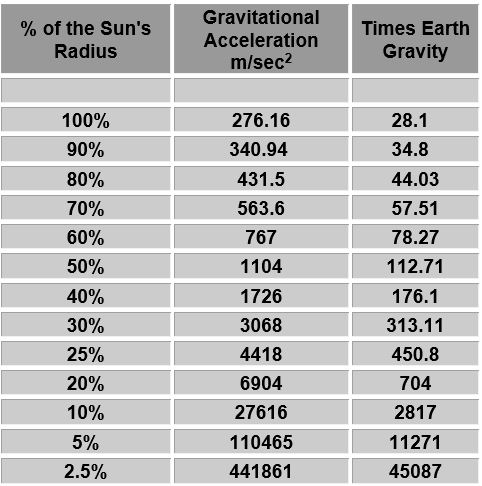Table: this table shows that how gravitational acceleration changing with change in radius ultimately change in density but it’s allow to the mass is remain static.

We know that according to Newton’s second law of motion, Force is the multiplication of mass and acceleration. So, if we change the acceleration will result change in force.     (F = ma) This law of force can be written combine with Newton’s gravitation law as-Now we can get the answer of our question. Although Newton’s law of gravitation claims the two forces will be same, the attraction force between A and C is greater than the attraction force between B and C. Because of the value of F = mg of star A for planet C is greater than the value of F = mg of star B for planet C though they have same mass. It is because there is difference between their gravitational acceleration ultimately means difference in density.  This problem is likely to occur when the mass is huge or there is a wide variation of density among objects.

From above it is prominent that there is another factor that is responsible for the gravitational force. We see that- due to change in density cause change in acceleration which is responsible for change of gravitational force. But there is a problem; density is related with mass by the following equation-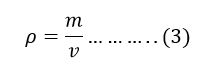Definition of Kilo-gram:

According to International System of Unit, the unit of mass is kilogram. The gram was originally defined in 1795 as the mass of one cubic centimeter of water at the melting point of water, making the kilogram equal to the mass of one liter of water. But it is difficult to measure water accurately. To overcome this problem International Prototype Kilo-gram is manufactured.  The original prototype kilogram, manufactured in 1799 and from which the IPK is derived, had a mass equal to the mass of 1.000025 liters of water at 4 °C. The IPK is made of a platinum alloy known as "Pt‑10Ir", which is 90% platinum and 10% iridium (by mass) and is machined into a right-circular cylinder.

From the definition of kilogram we can see that mass is measured by IPK which is equal to the one liter water. It means- everything is measured in the term of water which have a definite density. For example-take an object which have a mass of 50 kg. By 50 kg what can be understand if we don’t specified the object? Can we understand the density or volume of the object? In Newton Gravitation Law, the gravitation force is depends on masses and distance. But we have seen from the life cycle of star, attraction force of a star or celestial object is different in there different stage of their life cycle due to change in density though their mass remain unchanged.

To overcome this problem, a new factor Specific Gravity is taken. This factor is taken due to some reason as follow-

·         Specific gravity is the ratio of the density of a substance to the density of a reference substance. The reference substance is water.·         Since as everything measure in the term of water, it only indicate the density of the object.

·         It is a ratio of densities, is a dimensionless quantity. For this reason, the unit of force remain Newton(N)

EXAMPLE:

The Solar system comprises the Sun and the objects that orbit it, either directly or indirectly. Of those objects that orbit the Sun directly, the largest eight are the planets(Pluto is now consider as large object in Kuiper Belt) that form the planetary system around it, while the remainders are significantly smaller objects, such as dwarf planets and small Solar System bodies such as comets and asteroids.

All the planets directly orbit the sun and satellites directly orbit their planet by centripetal force. [Centripetal force is a force that makes a body follows a curved path. Its direction is always orthogonal the velocity of the body and towards the fixed point of the instantaneous center of curvature of the path.] This centripetal force is achieved from the gravitational attraction force. From above we can say. An object orbits that object which attract more strongly than any other object. Now think about our planet Earth and its satellite Moon. Earth orbits sun and moon orbits earth by centripetal force which is achieved from gravitational force.

According Newton’s Gravitational Law, the gravitation force between Earth and Moon is-

We know,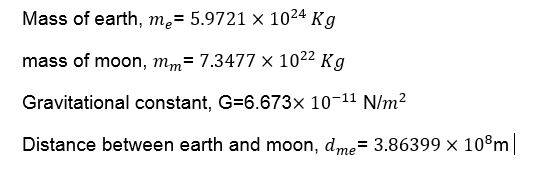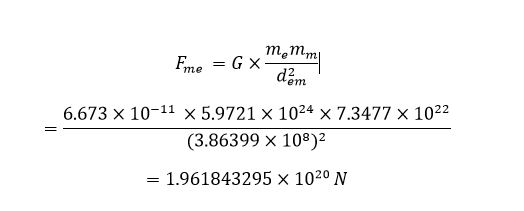According Newton’s Gravitational Law, the gravitation force between Sun and Moon is Again we know,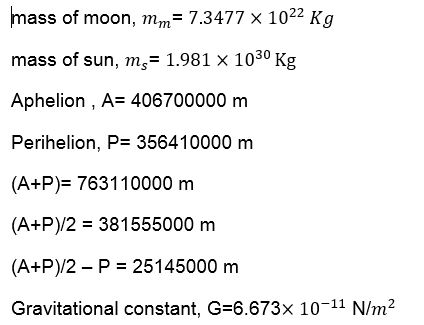So, the distance between moon and sun,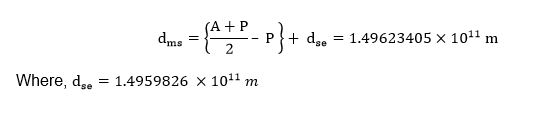[Note: this is because for more precise]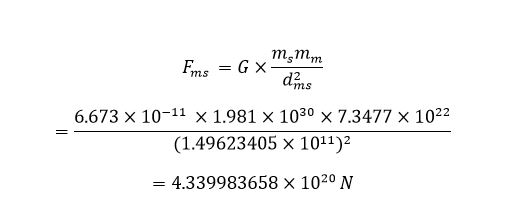From above calculation, it is clear that sun attract moon more strongly than earth which is slightly above of double (2.2121969).According to newton’s law moon should orbit sun but it don’t. Because of another factor that works behind of these which is specific gravity.

According to equation (1), the gravitation force between Earth and Moon is-

We know,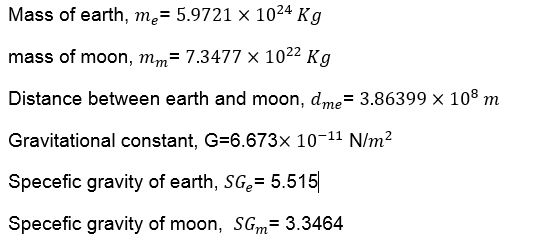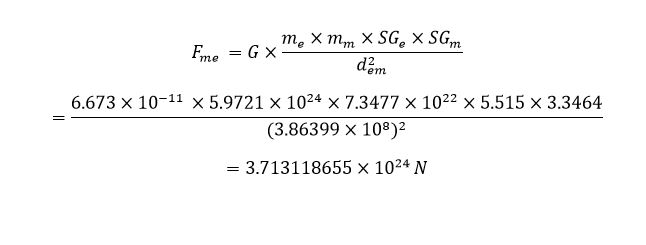According to equation (1), the gravitation force between Sun and Moon is-

Again we know,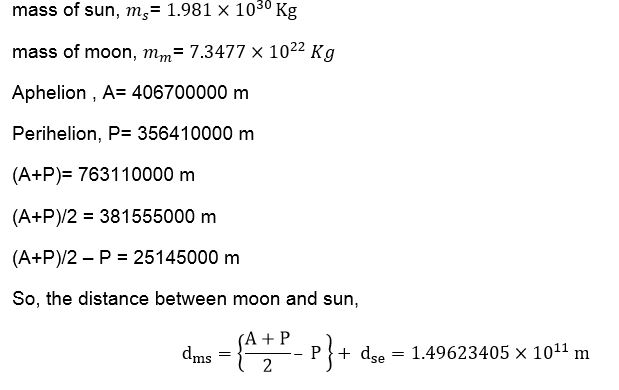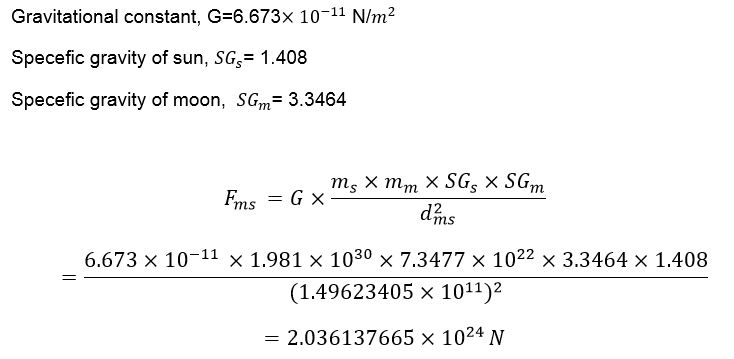DECITION:

From above calculation, it is clear that equation (1) is satisfactory for moon where Newton’s gravitation law is unable to describe. Equation (1) is not only satisfactory for moon but also all the planet. So, further research should be carried out

REFERENCE:

• A Brief History of Time - Stephen Hawking
• Newton's Philosophiae Naturalis Principia Mathematica
• The Michell-Cavendish Experiment, Laurent Hodges

·          Einstein's Theory of Relativity

·         Lewin, Newton’s First, Second, and Third Laws  Lecture 6. (6:53–11:06)

·         John Robert Taylor (2005). Classical Mechanics

·         http://www.columbia.edu/~ah297/unesa/sun/sun-chapter1.html

·         http://www.vcs.ethz.ch/chemglobe/ptoe/_/1.html